# AP Chemistry : Atomic Structure and Properties

## Example Questions

### Example Question #712 : Ap Chemistry

How many atoms are there inmoles of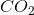?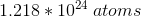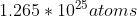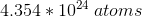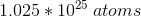Explanation:

This question is asking us to determine how many atoms there are inmoles of. Rather than asking for the number of molecules, our final answer will need to tell us the number of atoms. Since there areatoms for every molecule of, we'll first need to find out how many molecules there are.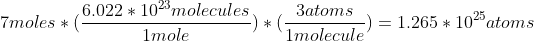### Example Question #1 : Mass Spectroscopy Of The Elements

A certain element X is comprised of isotopes A, B, and C. Isotope A has a mass of 50 amu and is 70% of naturally occurring X. Isotope B is 35 amu and is 25% of X. Isotope C has a mass of 70 amu and is 5% of X. What is the atomic weight of element X?

55 g/mol

47 g/mol

87 g/mol

70 g/mol

40 g/mol

47 g/mol

Explanation:

0.70 (50 amu) + 0.25 (35 amu) + 0.05 (70 amu) = 47 amu = 47 g/mol

### Example Question #2 : Mass Spectroscopy Of The Elements

The average molecular weight for element X is 59.97 g/mol. There are two known isotopes of element X, one weighing 59 g/mol, and the other weighing 61 g/mol. What is the relative abundance of each?

75% X-59, 25% X-61

51.5% X-59, 48.5% X-61

50% X-59, 50% X-61

48.5% X-59, 51.5% X-61

63% X-59, 37% X-61

51.5% X-59, 48.5% X-61

Explanation:

In this simplistic case, we only have two isotopes, so we know the following: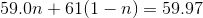Where "n" represents the relative abundance of X-59, and "(1-n)" is the relative abundance of X-61. We will algebraically solve for "n":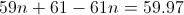simplifies to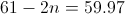simplifies to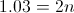which leads to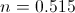If "n" is equal to 0.515, that means the relative abundance of X-59 is 51.5%, and thus the relative abundance of X-61 is 48.5%.

### Example Question #3 : Mass Spectroscopy Of The Elements

Arbitrary element, X, has two isotopes,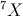and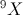. The mass listed on the periodic table is 8.000 grams per mole. Without doing any calculations, which isotope is more abundant?

They are both present in equal amounts

There must be a third isotope in order for this to occuris more prevalent

There is not enough information to answer this questionis more prevalent

They are both present in equal amounts

Explanation:

A calculation would show that the relative abundance of each is 50%, you can see that 8.000 g/mol is exactly the midpoint between the masses of 7 and 9, so each has to be present in equal quantities.

### Example Question #4 : Mass Spectroscopy Of The Elements

An element has two main isotopes. The first isotope has a molar mass of 125 grams, and the second isotope has a molar mass of 128 grams. A naturally occurring sample of the element has a molar mass of 126.8 grams.

What is the lighter isotope's percentage in the natural sample?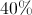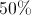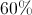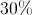Explanation:

In order to find the percentage of the lighter isotope in the natural sample, we need to use an equation that compares the percentage of each isotope and the natural molar mass. Since both of the isotopes together will account for 100% of the natural sample, we can say that the lighter isotope's percentage is, while the remaining percentage is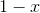.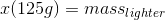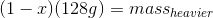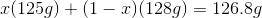Use this equation to solve for.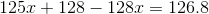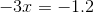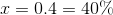In other words, we can conclude that the lighter isotope makes up 40% of the natural sample.

### Example Question #5 : Mass Spectroscopy Of The Elements

Chemical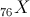has only two isotopes: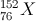and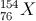. The atomic weight of chemical X, listed on the periodic table, is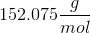. What is the relative abundance of each isotope?

There is not enough information to answer this question

The abundance ofis 96.25%, and the abundance ofis 3.75%

The abundance ofis 91.25%, and the abundance ofis 8.75%

The abundance ofis 3.75%, and the abundance ofis 96.25%

The abundance ofis 50%, and the abundance ofis 50%

The abundance ofis 96.25%, and the abundance ofis 3.75%

Explanation:

The abundance ofwill be referred to as "n," and the abundance ofis "1-n." Together, we know that these values account for the full abundance of chemical X in existence.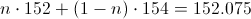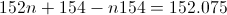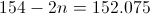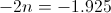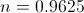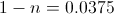The abundance ofis 96.25%, and the abundance ofis 3.75%.

### Example Question #6 : Mass Spectroscopy Of The Elements

Many elements exist in nature as varying isotopes. Although one isotope commonly predominates due to stability, the other isotopes can affect the overall mass of one natural mole of the element.

Suppose a mystery element has two main isotopes: isotope X has a molar mass of 128 grams per mole and isotope Y has a molar mass of 132 grams per mole. One mole of this element, when found naturally, weighs 128.8 grams. What are the percentages of isotopes X and Y when found in nature?

50% X and 50% Y

80% X and 20% Y

60% X and 40% Y

20% X and 80% Y

80% X and 20% Y

Explanation:

This answer becomes a lot easier if we use some quick mental math. First, notice that if the two isotopes were equal in nature (50/50), than a mole would weigh 130 grams.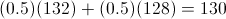Since the number is less than that, we can conclude that isotope X is more dominant than isotope Y, lowering the average mass. Now, we can use math to find the exact percentage.

Start by realizing that isotope X will make up some percentage of the element in nature (we will call this percentage "A") while isotope Y will make up the rest of that percentage (1-A).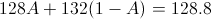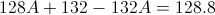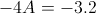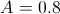This means that 80% of the element is composed of isotope X, and the other 20% is composed of isotope Y. Going back to our mental math, we see that, just as we predicted, isotope X is present in a larger amount than isotope Y.

### Example Question #7 : Mass Spectroscopy Of The Elements

Which of the following is not a subatomic particle?

Proton
Alpha particle
Neutron
Electron
Explanation:

Protons, neutrons, and electrons are all subatomic particles. Alpha particles consist of 2 protons and 2 neutrons bound together in a particle. It is usually emitted during fission reactions.

### Example Question #8 : Mass Spectroscopy Of The Elements

Which of the following statements is true?

Electrons are smaller in size than nucleons, but have equal charge

Electrons are larger than protons, which means they must have less charge than protons

The nucleus of an atom is electrically neutral

Electrons are smaller than protons, which means they have less charge than protons

Electrons are close in proximity to the atomic nucleus

Electrons are smaller in size than nucleons, but have equal charge

Explanation:

Electrons are notably smaller than nucleons (protons and neutrons), however, they have an equal amount of charge to protons. This is why an atom with the same amount of protons as electrons is considered electrically neutral.

The nucleus itself is positively charged, as the electrons are located relatively far away from the atomic center.

### Example Question #9 : Mass Spectroscopy Of The Elements

What is the sum of the number of neutrons of the following atoms: (a) N15
(b) Ag109 (c) Si30

84

88

90

86

82